Writing a Basic Analog Clock UserControl in Visual Basic.Net (Part 1)

Published on
19,529 Points
13,029 Views
5 Endorsements
Approved
Creating an analog clock UserControl seems fairly straight forward.  It is, after all, essentially just a circle with several lines in it!  Two common approaches for rendering an analog clock typically involve either manually calculating points with trigonometric functions, or rotating the entire drawing the surface to draw lines at specific angles.  This article will demonstrate just how easy it is to make an analog clock in VB.Net using the latter rotation method.

The “Bare Bones” Analog Clock: A Circle with Several Lines

Let’s begin by building a “bare bones” analog clock as described above: “a circle with several lines in it.”  Start by adding a new UserControl to your Project.  Click on Project --> Add User Control, change the name in the box to “AnalogClock” and press Enter to create it.  Most of our work will be done in the Paint() event so let’s start there.  With the UserControl selected, go to the Properties Pane and click on the “Lightning Bolt” icon to get a list of events.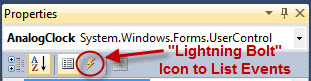Scroll down to the “Paint” entry and double click it.  You should now have this in your editor:
Public Class AnalogClock

Private Sub AnalogClock_Paint(ByVal sender As System.Object, ByVal e As System.Windows.Forms.PaintEventArgs) Handles MyBase.Paint

End Sub

End Class
The Paint() event supplies a Graphics surface via “e.Graphics” that allows us to draw on the UserControl.  In the .Net Framework, the default coordinate system places the origin at the upper left corner with x values increasing as you move to the right, and y values increasing as you move down.  It would be much nicer if we could instead treat the center of our analog clock as the origin, thus allowing us to easily rotate around the center of the clock.  This can be achieved using the Graphics.TranslateTransform() method.  The first order of business is to calculate the center point of the UserControl, which is computed by simply halving the width and height:
Dim center As New Point(Me.ClientSize.Width / 2, Me.ClientSize.Height / 2)
Next we move the origin by passing the X and Y values of our center point to TranslateTransform():
e.Graphics.TranslateTransform(center.X, center.Y)
Now the point (0, 0) is located at the center of our UserControl!  Let’s use this fact to draw the circle of our clock at the origin.  First we need to compute an appropriate radius for our circle by selecting the smaller value between the width and height of our control, and then taking a percentage of that:
Dim radius As Integer = Math.Min(Me.ClientSize.Width, Me.ClientSize.Height) / 2 * 0.8
I’ve used 0.8, or 80%, to ensure that our circle will always be completely visible and have a small margin between it and the edges of the control.  The next step is to create a bounding box that our circle will be drawn in.  We start with a rectangle located at (0, 0) and having a size of 1x1 pixel.  Then we use the Inflate() method and our computed radius to make the rectangle expand to our desired target size while still keeping the same center point (the origin).  Lastly, we render the circle with DrawEllipse():
Dim clock As New Rectangle(New Point(0, 0), New Size(1, 1))
e.Graphics.DrawEllipse(Pens.Black, clock)
At this point, the code in our analog clock control should look like this:
Public Class AnalogClock

Private Sub AnalogClock_Paint(ByVal sender As System.Object, ByVal e As System.Windows.Forms.PaintEventArgs) Handles MyBase.Paint
Dim center As New Point(Me.ClientSize.Width / 2, Me.ClientSize.Height / 2)
Dim radius As Integer = Math.Min(Me.ClientSize.Width, Me.ClientSize.Height) / 2 * 0.8
e.Graphics.TranslateTransform(center.X, center.Y)

Dim clock As New Rectangle(New Point(0, 0), New Size(1, 1))
e.Graphics.DrawEllipse(Pens.Black, clock)
End Sub

End Class
This should produce a circle in the center of our control no matter what its width or height is.  To verify this, in the menus, click on Build --> Build Solution.  Once compiled, double click on your default Form in Solution Explorer to go back to design mode.  You should now find a new UserControl called “AnalogClock” at the top of your Toolbox: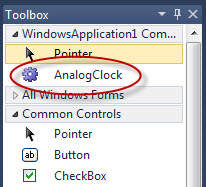Select the AnalogClock control in the Toolbox and drag a new one somewhere on your form.  Grab one of its corners and drag it around to see what happens as the control is resized.  Does the circle stay centered and adjust its size accordingly?  Hopefully you answered with, “Yes!”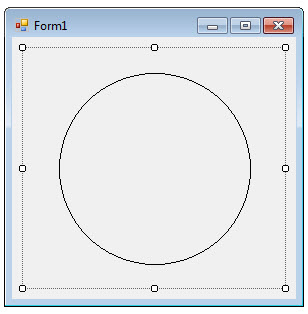All that is left to do now is draw the hour, minute, and second hands of our clock.  At the beginning of the article I stated that I would draw the clock by rotating the graphics surface instead of using trigonometric functions.  What does that really mean though?  Specifically, I will draw all three hands of the clock as horizontal lines on the x-axis, extending from the origin and to the right.  They will appear in different locations, though, because we will literally rotate around the center of the clock and move the x-axis to where we want it!

How will we know what angle to use for each hand?  Start with the fact that a circle has 360 degrees.  In the .Net framework, 0 (zero) degrees is on the x-axis pointing to the right.  Increasing the value of our angle moves us in a clockwise direction.  This would put 90 degrees pointing straight down on the y-axis, 180 degrees pointing left on the x-axis, and 270 degrees pointing straight up on the y-axis.  A positive increase in angle moves us clockwise, while a negative decrease in angle moves us counter-clockwise.  Thus 45 degrees is pointing down and to the right halfway between the X and Y axis, while -45 degrees is pointing up and to the right halfway between the X and Y axis.

A standard clock has 12 hour positions on it.  If we divide 360 degrees by 12, we get 30 degrees.  This is how many degrees are between each hour position on the clock face.  The following video sweeps through the angles from 0 to 360, in both the positive and negative directions, showing the multiples of 30 and 45 degrees as red dashed lines:  Idle-Mind-449853.flv
With a clear understanding of the angle system in .Net, we can now move on to computing angles from the current time at which to draw the hands of our analog clock.  The current time can be retrieved using the DateTime.Now function.  From that return value, we can extract the hour, minute, and second values using the respective Hour, Minute, and Second properties.  Let’s deal with the hour value first.  The Hour property returns a value between 0 and 23.  Values less than or equal to 12 are AM times, and values greater than or equal to 13 are PM times.  Since an analog clock only has positions between 1 and 12, we must convert all PM times (which are greater than 12) to AM times by subtracting 12 from them.  This is an exercise quite familiar to anyone accustomed to converting between military and standard times.  The conversion from a 24 hour to a 12 hour value can be accomplished with the below line of code:
Dim Hour As Integer = IIf(DateTime.Now.Hour >= 12, DateTime.Now.Hour - 12, DateTime.Now.Hour)
The IIf() function evaluates the first parameter and returns the second parameter if the first is true, or the third parameter if the first is false.  Note that 12 in the afternoon becomes a zero, since 12 minus 12 equals zero.  Experienced programmers will immediately point out that this can be accomplished with much shorter code using the Mod function:
Dim Hour As Integer = DateTime.Now.Hour Mod 12
Use whatever you’re more comfortable with, as both versions are valid.  One will likely make your eyebrow rise like Dr. Spock, while the other will magically seem to just “make more sense”.  With the Hour component scaled down to values between 0 and 11, we can compute what percentage of 360 it represents:
Dim HourAngle As Integer = Hour / 12 * 360
Pretend the time is currently 1:00 PM.  The Hour value would return 13, which we scale down to 1.  Next we divide 1 by 12, and multiply that by 360 to get the angle of 30.  A value of 30 degrees would be pointing down and to the right with respect to the center of our control (see the angles video above).  Unfortunately, this isn’t where the hour hand should be on an analog clock for 1:00 PM!  To get the hour hand at the correct angle, we have to rotate it 90 degrees in the counter-clockwise direction.  This is done by subtracting 90 from the previous calculation making the correct formula for the angle of the hour hand:
Dim HourAngle As Integer = Hour / 12 * 360 – 90
The following lists the correct angles for all 24 hours as computed by the above formula:
Hours | Angle
0,12 | -90
1,13 | -60
2,14 | -30
3,15 |  0
4,16 |  30
5,17 |  60
6,18 |  90
7,19 |  120
8,20 |  150
9,21 |  180
10,22 |  210
11,23 |  240
To rotate the graphics surface, we simply pass the angle to rotate by to the Graphics.RotateTransform() method.  Keep in mind that the value passed in is not an absolute value, but a relative one.  It instructs the graphics surface to rotate in the clockwise (positive angle) or counter-clockwise (negative angle) direction by the amount passed in.  If you need the rotation to be absolute then you must ensure that the graphics was at zero degrees to begin with.  This can be done by either resetting the surface with Graphics.ResetTransform(), or by rotating the graphics backwards by the opposite amount of any previous rotation.  If you use ResetTranform() don’t forget to move the origin back to your desired center with TranslateTransform() before calling RotateTransform() again.  So to rotate our surface in preparation for drawing the hour hand we would use this line of code:

e.Graphics.RotateTransform(HourAngle)
Now that we have the angle computed and the surface rotated, let’s actually draw the hour hand on our clock.  Previously I had stated that I would draw all the hands as horizontal lines on the x-axis, extending from the origin and to the right.  So the line will start at (0, 0) and extend to some point on the right (x, 0).  The length of the hand should obviously be less than the radius of our circle, but what is a good value?  Just as we calculated the radius as a percentage of the UserControl size, our hand length can be calculated as a percentage of the radius.  It should be less than radius but allow enough room for the minute hand to be longer.  On most clocks the hour hand is shorter than the minute hand, so I will use 65% for the hour hand and 80% for the minute and second hands.  Here is the code I used to calculate the length of the hour hand and draw it on the clock face in red:
x = radius * 0.65
e.Graphics.DrawLine(Pens.Red, 0, 0, x, 0)
Since the surface had already been rotated, drawing on the x-axis to the desired length will make the line appear at the correct angle instead of as a horizontal line (unless the current hour angle happened to be zero).  In preparation for drawing the next hand of the clock, I immediately undo the rotation by passing in the opposite of HourAngle to RotateTransform().  This will leave the graphics surface at a net rotation of zero degrees:
e.Graphics.RotateTransform(-HourAngle)
Put all together, the code for computing the angle, rotating the surface, and drawing the hour hand is:
x = radius * 0.65
Dim Hour As Integer = IIf(DateTime.Now.Hour >= 12, DateTime.Now.Hour - 12, DateTime.Now.Hour)
Dim HourAngle As Integer = Hour / 12 * 360 - 90
e.Graphics.RotateTransform(HourAngle)
e.Graphics.DrawLine(Pens.Red, 0, 0, x, 0)
e.Graphics.RotateTransform(-HourAngle)
The code for drawing the minute and seconds hands is very similar except that we don’t have to do any scaling of the values before we use them.  Simply compute the percentage of 60 that the current minute/second value is and multiply that by 360.  There are six degrees between each position of the minute/second hands.  Just as with the hour hand, we still need to offset it by -90 degrees so that zero is pointing straight up instead of to the right.  I draw the minute hand in blue and the second hand in black.  Since the hour hand is shorter than the minute hand, we should draw the minute hand first and the hour hand second so that it will be visible when they are the same value.  Here is the complete Paint() event code that draws the clock circle along with hour, minute, and second hands:
Private Sub BareBonesAnalogClock_Paint(ByVal sender As Object, ByVal e As System.Windows.Forms.PaintEventArgs) Handles Me.Paint
Dim center As New Point(Me.ClientSize.Width / 2, Me.ClientSize.Height / 2)
Dim radius As Integer = Math.Min(Me.ClientSize.Width, Me.ClientSize.Height) / 2 * 0.8
e.Graphics.TranslateTransform(center.X, center.Y)

' the clock circle
Dim clock As New Rectangle(New Point(0, 0), New Size(1, 1))
e.Graphics.DrawEllipse(Pens.Black, clock)

' draw the minute hand
Dim x As Integer = radius * 0.8
Dim MinuteAngle As Integer = DateTime.Now.Minute / 60 * 360 - 90
e.Graphics.RotateTransform(MinuteAngle)
e.Graphics.DrawLine(Pens.Blue, 0, 0, x, 0)
e.Graphics.RotateTransform(-MinuteAngle)

' draw the hour hand
x = radius * 0.65
Dim Hour As Integer = IIf(DateTime.Now.Hour >= 12, DateTime.Now.Hour - 12, DateTime.Now.Hour)
Dim HourAngle As Integer = Hour / 12 * 360 - 90
e.Graphics.RotateTransform(HourAngle)
e.Graphics.DrawLine(Pens.Red, 0, 0, x, 0)
e.Graphics.RotateTransform(-HourAngle)

' draw the second hand
x = radius * 0.8
Dim SecondAngle As Integer = DateTime.Now.Second / 60 * 360 - 90
e.Graphics.RotateTransform(SecondAngle)
e.Graphics.DrawLine(Pens.Black, 0, 0, x, 0)
e.Graphics.RotateTransform(-SecondAngle)
End Sub
Recompile the UserControl by hitting Build --> Build Solution again and the AnalogClock control on your form should now look something like this: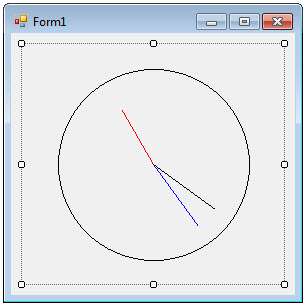We just need to add one last component to our control to make it complete.  In its current state, the clock is static and doesn’t update with the current time.  Ideally, the clock should refresh every second to make the hands move properly.  This can be accomplished by adding a Timer() control to our code and handling the Tick() event.  First, let’s declare a new instance of the Timer() control as WithEvents at the class level:
Private WithEvents Tmr As New System.Windows.Forms.Timer
Next, across the top of the code editor, change the left dropdown to “Tmr” and the right dropdown to “Tick”.  This should add the blank stub event handler shown below; to which I’ve added one line of code, “Me.Refresh()”, to make the UserControl repaint itself whenever the Tick() event fires:
Private Sub Tmr_Tick(ByVal sender As Object, ByVal e As System.EventArgs) Handles Tmr.Tick
Me.Refresh()
End Sub
For the Timer to do its job properly we need to set its Interval() property and turn it on.  One good place to do this from would be the Load() event of the UserControl which fires before the control becomes visible for the first time.  To get this event, across the top of the code editor, change the left dropdown to “(AnalogClock Events)” and the right dropdown to “Load”.  In the stub inserted for me, I set the Timer Interval to 1000 to make it fire once every second, and called the Start() method to make it start firing:
Private Sub AnalogClock_Load(ByVal sender As Object, ByVal e As System.EventArgs) Handles Me.Load
Tmr.Interval = 1000
Tmr.Start()
End Sub
That’s it!  We now have a working analog clock UserControl that shows the correct time.  Here is the complete code listing for the “bare bones” version of the control:
Public Class AnalogClock

Private WithEvents Tmr As New System.Windows.Forms.Timer

Private Sub AnalogClock_Load(ByVal sender As Object, ByVal e As System.EventArgs) Handles Me.Load
Tmr.Interval = 1000
Tmr.Start()
End Sub

Private Sub Tmr_Tick(ByVal sender As Object, ByVal e As System.EventArgs) Handles Tmr.Tick
Me.Refresh()
End Sub

Private Sub AnalogClock_Paint(ByVal sender As Object, ByVal e As System.Windows.Forms.PaintEventArgs) Handles Me.Paint
Dim center As New Point(Me.ClientSize.Width / 2, Me.ClientSize.Height / 2)
Dim radius As Integer = Math.Min(Me.ClientSize.Width, Me.ClientSize.Height) / 2 * 0.8
e.Graphics.TranslateTransform(center.X, center.Y)

' the clock circle
Dim clock As New Rectangle(New Point(0, 0), New Size(1, 1))
e.Graphics.DrawEllipse(Pens.Black, clock)

' draw the minute hand
Dim x As Integer = radius * 0.8
Dim MinuteAngle As Integer = DateTime.Now.Minute / 60 * 360 - 90
e.Graphics.RotateTransform(MinuteAngle)
e.Graphics.DrawLine(Pens.Blue, 0, 0, x, 0)
e.Graphics.RotateTransform(-MinuteAngle)

' draw the hour hand
Dim Hour As Integer = IIf(DateTime.Now.Hour >= 12, DateTime.Now.Hour - 12, DateTime.Now.Hour)
Dim HourAngle As Integer = Hour / 12 * 360 - 90
e.Graphics.RotateTransform(HourAngle)
x = radius * 0.65
e.Graphics.DrawLine(Pens.Red, 0, 0, x, 0)
e.Graphics.RotateTransform(-HourAngle)

' draw the second hand
Dim SecondAngle As Integer = DateTime.Now.Second / 60 * 360 - 90
x = radius * 0.8
e.Graphics.RotateTransform(SecondAngle)
e.Graphics.DrawLine(Pens.Black, 0, 0, x, 0)
e.Graphics.RotateTransform(-SecondAngle)
End Sub

End Class
Admittedly, this is a pretty unimpressive clock!  The control may not be immediately recognizable as an analog clock, and it’s hard to read.  It is true, though, to the original description of “a circle with several lines in it”.  In the next article, we will transform this “bare bones” control into an analog clock that is actually usable in a project: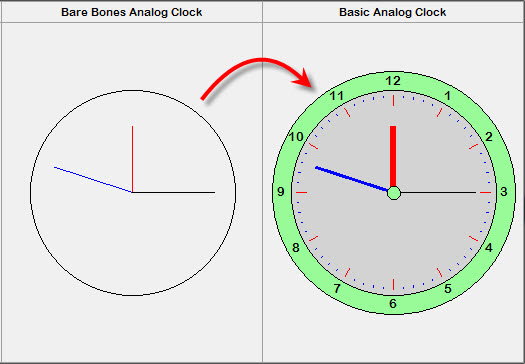5